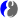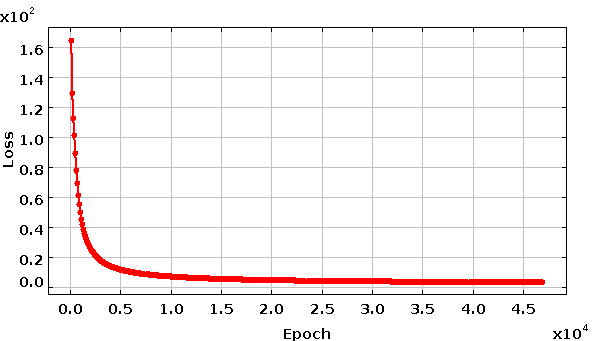# DMelt:AI/5 Convolutional NN

Limitted access. First login to DataMelt if you are a full DataMelt member. Then login to HandWiki as a user.

# Convolutional NN

Convolutional neural network (CNN) is comprised of one or more convolutional layers and then followed by one or more fully connected layers as in a standard multilayer neural network. The CNN are effective in areas such as image recognition and classification. ConvNets have been successful in identifying faces, objects and traffic signs apart from powering vision in robots and self driving cars.

Convolutional networks in DataMelt can be found in these Java packages:

To run the examples below, you will need to download the most recent , unpack it and run the script either in batch mode (dmelt_batch.sh example.py) or in the GUI mode (dmelt.sh example.py), assuming you are using Linux/Mac.

# Image format for CNN

In our example we will use images in theNetpbm format grayscale format (PGM). The name "PGM" is an acronym derived from "Portable Gray Map". The cell values range from 0 - 255. The files are in the binary format (it has the magic value "P5" - you can see it by opening one of such files). You can convert it to "Plain" (or uncompressed) PGM, where each pixel in the raster is represented as an ASCII decimal number (of arbitrary size). Use ImageMagic to do this transform:

convert input.pgm -compress None output.pgm

# CNN for image identification

We will consider an example which attempts to identify images with human faces using the JavaCNN package created by D.Persson. The package provides a simple Java API for CNN without external dependencies. Our input images are from a publicly available database . Let us copy a zip file with this (slightly modifies) database and unzip it. Create a file "example.py" and run these commands using DataMelt:

from jhplot import *
http='http://datamelt.org/dmelt/examples/data/'
print Web.get(http+"mitcbcl_pgm_set2.zip")
print IO.unzip("mitcbcl_pgm_set2.zip")

The last command unzips 2 directories "train" and "test". Each directory has images with faces ("face_*") and some other images ("cmu_*). Note that "_" in the file name is important since this will help us in the future to identify image type. The train directory has 1499 files with images of faces and 13673 files with other images.

Let us look at one image and study its properties using Java API as described in Sect. Using ImageJ. We will use theIJ package Java package.

from ij import *
imp = IJ.openImage("mitcbcl_pgm_set2/train/face_00001.pgm")
print "Width:", imp.width," Hight:", imp.height
imp.show()  # show this image in a frame
ip = imp.getProcessor().convertToFloat()
pixels = ip.getPixels()  # get array of pixels
print pixels  # print array with pixels

This code shows the image, print its size (19x19) and the pixel map. Now let us create a code which will do the following:

1. Reads the images from "train" directory
2. Reads the images from "test/" directory
3. Initialize the CNN and runs over 50 iterations
4. During each iteration it calculates the probability for correct identification images with faces from the "test/" directory and saves the CNN to a file
5. At the end of the training, it reads the trained CNN from the file and performs the final run over test images, printing the predictions.

Copy these lines and save in a file "example.py" and run inside the DataMelt:

# This example reads external file with images and uses JavaCNN to
# identify images with faces.
from jhplot import *
print Web.get("http://datamelt.org/examples/data/mitcbcl_pgm_set2.zip")
print IO.unzip("mitcbcl_pgm_set2.zip")

NMax=50 # Total runs. Reduce this number to get results faster
from org.ea.javacnn.data import DataBlock,OutputDefinition,TrainResult
from org.ea.javacnn.layers import DropoutLayer,FullyConnectedLayer,InputLayer,LocalResponseNormalizationLayer
from org.ea.javacnn.layers import ConvolutionLayer,RectifiedLinearUnitsLayer,PoolingLayer
from org.ea.javacnn.losslayers import SoftMaxLayer
from org.ea.javacnn import JavaCNN
from java.util import ArrayList,Arrays
from java.lang import System

layers = ArrayList(); de = OutputDefinition()
print "Total number of runs=", NMax
print "Total number of trainning images=",mr.size()," Nr of types=",mr.numOfClasses()
print "Total number of test images=",mrTest.size()," Nr of types=",mrTest.numOfClasses()
modelName = "model.ser" # save NN to this file

layers.add(ConvolutionLayer(de, 5, 32, 1, 2)) # uses different filters
layers.add(RectifiedLinearUnitsLayer())       # applies the non-saturating activation function
layers.add(PoolingLayer(de, 2,2, 0))          # creats a smaller zoomed out version

print "Training.."
net = JavaCNN(layers)

from jarray import zeros
numberDistribution,correctPredictions = zeros(10, "i"),zeros(10, "i")

start = System.currentTimeMillis()
db = DataBlock(mr.getSizeX(), mr.getSizeY(), 1, 0)
for j in range(NMax):
loss = 0
for i in range(mr.size()):
loss = loss + tr.getLoss()
if (i != 0 and i % 500 == 0):
print "Nr of images: ",i," Loss: ",(loss/float(i))
print "Loss: ", (loss / float(mr.size())), " for run=",j
mr.reset()
print 'Wait.. Calculating predictions for labels=', mr.getLabels()
Arrays.fill(correctPredictions, 0)
Arrays.fill(numberDistribution, 0)
for i in range(mrTest.size()):
net.forward(db, False)
prediction = net.getPrediction()
if(correct == prediction): correctPredictions[correct] +=1
numberDistribution[correct] +=1
mrTest.reset()
print " -> Testing time: ",int(0.001*(System.currentTimeMillis() - start))," s"
print " -> Current run:",j
print net.getPredictions(correctPredictions, numberDistribution, mrTest.size(), mrTest.numOfClasses())
print " -> Save current state to ",modelName
net.saveModel(modelName)

print "Read trained network from ",modelName," and make the final test"
Arrays.fill(correctPredictions, 0)
Arrays.fill(numberDistribution, 0)
for i in range(mrTest.size()):
net.forward(db, False)
prediction = net.getPrediction()
if(correct == prediction): correctPredictions[correct] +=1
numberDistribution[correct] +=1
print "Final test:"
print net.getPredictions(correctPredictions, numberDistribution, mrTest.size(), mrTest.numOfClasses())

50 iterations usually take a few hours. The final probability to identify images with human faces will be close to 85%.

# CNN for multiple image identification

In the example above, there were only 2 types of images (with faces and non-faces). You can use the same program as above to identify multiple image types. To run such example, you need to use the input file "mitcbcl_pgm_set1.zip" in the example of the previous section. The complete example is given here:

# Complete example for MNIST database

This example trains a CNN on the MNIST digits dataset  with 28x28 MNIST images. The dataset consists of handwritten digit images and it is divided in 60,000 examples for the training set and 10,000 examples for testing.All digit images have been size-normalized and centered in a fixed size image of 28 x 28 pixels. In the original dataset each pixel of the image is represented by a value between 0 and 255, where 0 is black, 255 is white and anything in between is a different shade of grey. The CNN crops a random 24x24 window before training on it (this technique is called data augmentation and improves generalization). Similarly to do prediction, 4 random crops are sampled and the probabilities across all crops are averaged to produce final predictions. Then it plots in real time losses vs iterations.

The processing takes quite a while, so you can stop it when the looses are small (and predicted rate is high). After each iteration, the neural network saves itself into a file. You can restore it and use it for predictions.

The output of the above script is shown below.# CNN using 2D arrays

In this example we will consider a Jython code which classifies 2D arrays using the CNN. First let us get the input data as. We will download MNIST test sample and unzip it in the current directory:

from jhplot import *
http='http://datamelt.org/examples/data/'
print Web.get(http+"mnist.zip")
print IO.unzip("mnist.zip")

The sample has twoASCII files: one for training and one for testing. Each file has multiple 2D arrays. The last element of the "feature" of this array, i.e. it labels the array. The goal is to build a CNN that uses the arrays from "train" file, and then calculate the prediction from "test" file. Here is a code:

We have chosen to use 10 iterations (takes a few minutes), which give very good probability for correct prediction (>95% cases for success). Increase this value to get larger rate of correct predictions.

1. https://github.com/kalaspuffar/JavaCNN
2. CBCL Face Database #1, MIT Center For Biological and Computation Learning